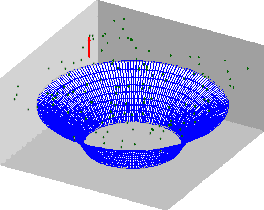# Bathtub vortex

Up to Case Studies

A bathtub vortex gives an ideal illustration to vorticity dynamics, which explains why when the water flows out, part of the angular momentum remains in the bath.When water flows through a hole in the bottom of the bath, it starts to rotate fast, as we all observed. Vortices that sometimes  appear near the air intakes of airliners before take-off and tornadoes are related phenomena, which occur when the fluid is being sucked into a localized area, be it the tab in a bath, air intake, or a hot air jet raising up in the surrounding colder air, as it happens in a tornado.

Why does the fluid rotate so fast?

In such situations the rotation is accelerated because of the conservation of angular momentum. Angular momentum is the product of mass, angular velocity and the square of the distance to the axis of rotation. When a figure skater makes a spin, she spreads her arms and starts the rotation. She than brings the arms in close to her body. This decreases their distance to the axis of rotation, and the angular momentum can stay the same only if the angular velocity increases. The same happens when fluid particles from far away approach the bath tub.

Where does the angular momentum come from?

This mechanism works only if the fluid has a non-zero angular momentum.

1. If the water is perfectly still before the tub is opened, it rotates with the Earth, and this alone might be enough. Equivalently, in the frame of reference linked to Earth, the initial appearance of the angular momentum is explained by the Coriolis force. However, a simple calculation shows that the resulting rotation will not be as strong as we often observe.

2. Another explanation is that normally when the plug is removed, water still have some angular momentum left from the time when the bath was filled with water.

3. If the bath is not symmetric, once the water starts moving, the bath walls can exert a force changing the angular momentum.

4. Even in a symmetric bath, the forces on the walls can also exert a non-symmetric force that changes the angular momentum. In that case, when the motion starts, it can eventually result in a clockwise or counter-clockwise rotating flow depending on a very small random initial perturbation, which grows due to the flow instability. Then, the faster the fluid rotates, the stronger the wall pushes it into more rotation.

All these four mechanisms are valid and can all be at work at the same time.

Does the angular momentum flow out?

Eventually, it does flow out with the water. Remarkably, however, over a certain, and might be quite long, period, water flows out but leaves part of its momentum behind. To understand how it works look at the animation. The red arrow shows a vorticity vector. Vorticity is advected by the fluid: this is why the vector goes around the axis together with the fluid particles. Vorticity is also rotated and stretched by the fluid as if it were an element of the material line. Fluid particles near the surface stay near the surface, but the fluid particles below the surface move down. This is why the vector is stretching as it approaches the axis of rotation. Due to the action of viscosity, vorticity is also diffused from the areas where it is large to the areas where it is small. As you can see from the animation, because of vorticity stretching in the bathtub vortex case this direction is outwards, away from the rotation axis. And vorticity is, as we know, the angular velocity of fluid particles.

Hence, one can say that while water flows out, it leaves part of the angular momentum back in the bath, thus adding to the rotation.

To get full insight into this interesting phenomenon, one can read the paragraph entitled “The intensification of vorticity by extension of vortex lines” in section 5.2 of the book by G. K. Batchelor, An Introduction to Fluid Dynamics (DOI:http://dx.doi.org/10.1017/CBO9780511800955), and the paper by J. Mizushima, K. Abe, and N.Yokoyama, Bathtub vortex induced by instability, in Physical Review E 90, 041002(R) (2014) (DOI:http://dx.doi.org/10.1103/PhysRevE.90.041002).

Next case study: Kelvin-Helmholtz instability.